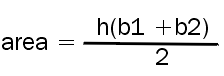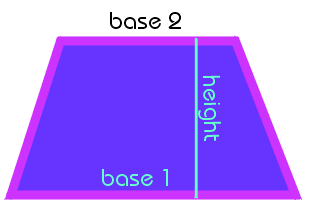A trapezoid has similar features to both a parallelogram and a triangle. It has one set of parallel sides.
 The sum of the two parallel sides times the height of the figure all divided by two will yield the area of the trapezoid.For the trapezoid on the right the area is 12(10 +15)/212(25)/2 = 300 cm^2base 1 = 15 cm, base 2 = 10 cm, the height = 12 cm

Find the area of the trapezoid
with these dimensions:
 Height Base 1 Base 2 14 12 30